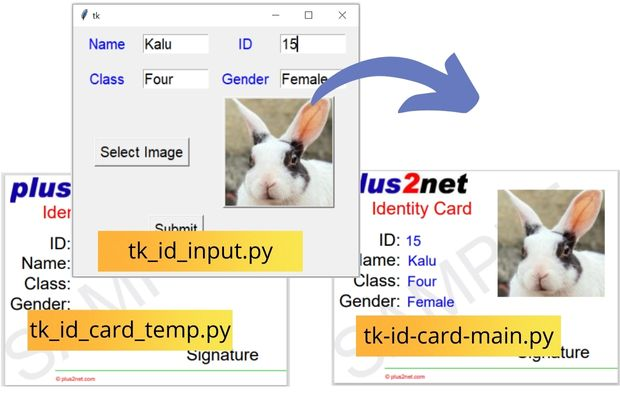# User inputs from Tkinter to create ID CardGenerating PDF ID card by using user input data & image through Tkinter window using ReportLab

## Tkinter window to take user inputs

We need five input data from user to create the ID Card.

`s_name` : Student name
`s_id` : Student ID
`s_class` : Student Class
`s_gender` : Student Gender
`s_filename` : Student picture as Path to the file.

We used StringVar() to collect data and pass the same to main file.
Button b2 is used to add click event to close the window ` command=lambda:my_w.destroy()`. Here is the full code.

tk_id_input.py
``````import tkinter as tk
from tkinter import filedialog
from PIL import Image, ImageTk
my_w = tk.Tk()
my_w.geometry("400x350")
s_name=tk.StringVar(value='Kalu')
s_id=tk.StringVar(value='16')
s_class=tk.StringVar(value='Four')
s_gender=tk.StringVar(value='Female')
s_filename=''
#def my_input():
l1=tk.Label(my_w,text='Name',font=22,width=8,fg='blue')
e1=tk.Entry(my_w,font=22,width=8,textvariable=s_name)

l2=tk.Label(my_w,text='ID',font=22,width=8,fg='blue')
e2=tk.Entry(my_w,font=22,textvariable=s_id,width=8)

l3=tk.Label(my_w,text='Class',font=22,width=8,fg='blue')
e3=tk.Entry(my_w,font=22,textvariable=s_class,width=8)

l4=tk.Label(my_w,text='Gender',font=22,width=8,fg='blue')
e4=tk.Entry(my_w,font=22,textvariable=s_gender,width=8)

b1 = tk.Button(my_w, text='Select Image',font=22,
b1.grid(row=2,column=0,columnspan=2)

global img,s_filename
f_types = [('Jpg Files', '*.jpg')]
img = ImageTk.PhotoImage(file=s_filename)
b4 =tk.Button(my_w,image=img) # using Button
b4.grid(row=2,column=2,columnspan=2)
#print(s_filename)

b2=tk.Button(my_w,text='Submit',font=22,command=lambda:my_w.destroy())

my_w.mainloop()``````

## Creating the ID card using data

We Import the blank template and use the same for creating the ID card.
``from tk_id_card_temp import my_temp # import the template``
Inside our main file tk_id_card_main.py we receive the five data from the above Tkinter window.
``from tk_id_input_v import s_name,s_id,s_class,s_gender,s_filename``
These variables are StringVar() so we will use get() method to collect the data and use them for creating the ID card . Full code is here

tk_id_card_main.py
``````from reportlab.pdfgen import canvas
import tkinter as tk

my_path='G:\\My drive\\testing\\pypdf2\\my_pdf.pdf' # update the path
from reportlab.lib.units import inch

from tk_id_card_temp import my_temp # import the template
from tk_id_input_v import s_name,s_id,s_class,s_gender,s_filename #tkinter

## comment below values if you are using above Tkinter window
#s_filename='D:\\images\\rabbit_face2.jpg'

c = canvas.Canvas(my_path,pagesize=(400,300))
c=my_temp(c) # run the template

c.setFillColorRGB(0,0,1)
c.setFont("Helvetica", 20)
c.drawString(0.5*inch,1.7*inch,str(s_id.get()))
c.drawString(0.5*inch,1.3*inch,s_name.get())
c.drawString(0.5*inch,0.9*inch,s_class.get())
c.drawString(0.5*inch,0.5*inch,s_gender.get())
######
c.showPage()
c.save()``````
We used one template by adding logo, labels , line and some copyright text. We disused about the blank template in Part 1. Full code is here

tk_id_card_temp.py
``````from reportlab.lib.units import inch
def my_temp(c):
c.translate(inch,inch)
c.setFont("Helvetica",14)

c.setStrokeColorRGB(0.1,0.8,0.1)
c.setFillColorRGB(0,0,1)
c.drawImage('D:\\top2.jpg',-0.9*inch,2.6*inch)
#####
c.rotate(35)
c.setFillColorCMYK(0,0,0,0.08) # font colour
c.setFont("Helvetica", 100)
c.drawString(-1.1*inch, -0.5*inch, "SAMPLE") # watermarking
c.rotate(-35)
#####
c.setFillColorRGB(1,0,0)
c.setFont("Helvetica", 25)
c.drawRightString(1.7*inch,2.3*inch,'Identity Card')
######
c.setFillColorRGB(0,0,0)
c.setFont("Helvetica", 24)
c.drawRightString(0.3*inch,1.7*inch,'ID:')
c.drawRightString(0.3*inch,1.3*inch,'Name:')
c.drawRightString(0.3*inch,0.9*inch,'Class:')
c.drawRightString(0.3*inch,0.5*inch,'Gender:')
c.drawRightString(4.0*inch,-0.5*inch,'Signature')
#####
c.line(-1.1,-0.7*inch,5*inch,-0.7*inch)
c.setFont("Helvetica",8)
c.setFillColorRGB(1,0,0) # font colour
c.drawString(0, -0.9*inch, u"\u00A9"+" plus2net.com")
####
return c``````

Subscribe to our YouTube Channel here

## Subscribe

* indicates required
Subscribe to plus2netplus2net.com